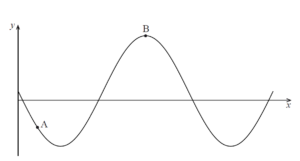# IBDP Past Year Exam Questions – Application of Differentiation

###### Q1.  [N08.P1]- 7 marks

Find the equation of the normal to the curve $5x{y}^{2}–2{y}^{2}=18$ at the point (1, 2) .

###### Q2.   [N09.P1]- 7 marks

A certain population can be modelled by the differential equation ,  where $y$ is the population at time $t$ hours and $k$ is a positive constant.
(a)  Given that $y={y}_{0}$ when $t=0$ , express $y$ in terms of $k$ , $t$ and ${y}_{0}$ .
(b) Find the ratio of the minimum size of the population to the maximum size of the population.

###### Q3.   [M09.P1]-5 marks

The diagram below shows a curve with equation , defined for $0\le x\le 3\mathrm{\pi }$ .The point lies on the curve and B $\left(a,b\right)$ is the maximum point.
(a) Show that $k=–6$ .
(b) Hence, find the values of $a$ and $b$ .                                  [3 marks]

###### Q4.   [N10.P1]- 8 marks

Consider the curve $y=x{e}^{x}$ and the line $y=kx,k\in R$ (a) Let $k=0$ .

(l)Show that the curve and the line intersect once.

(ll)Find the angle between the tangent to the curve and the line at the point of intersection.

(b)Let $k=1$ . Show that the line is a tangent to the curve.

###### Q5.   [M10.P1]- 8 marks

The function f is defined by $f\left(x\right)={e}^{{x}^{2}–2x–1.5}$ .

(a) Find ${f}^{‘}\left(x\right)$ .

(b) You are given that $y=\frac{f\left(x\right)}{x–1}$ has a local minimum at $x=a$ , $a>1$ . Find the value of $a$ .

###### Q6.   [M14.P1]- 9 marks

A curve has equation $arc\mathrm{tan}{x}^{2}+arc\mathrm{tan}{y}^{2}=\frac{\mathrm{\pi }}{4}.$

(a) Find $\frac{dy}{dx}$ in terms of $x$  and $y$ .

(b) Find the gradient of the curve at the point where $x=\frac{1}{\sqrt{2}}$ and

$y<0$ .

###### Q7.   [N14.P1]- 6 marks

A tranquilizer is injected into a muscle from which it enters the bloodstream. The concentration   $C$ in $mg{l}^{–1}$ , of tranquilizer in the bloodstream can be modelled by the function $C\left(t\right)=\frac{2t}{3+{t}^{2}}$ , $t\ge 0$ where $t$ is the number of minutes after the injection. Find the maximum concentration of tranquilizer in the bloodstream.

###### Q8.   [M15.P1]- 8 marks

In triangle $ABC$ ,   $BC=\sqrt{3}cm$ , $A\stackrel{^}{B}C=\theta$ and $B\stackrel{^}{C}A=\frac{\mathrm{\pi }}{3}$ .

(a) Show that length .

(b) Given that $AB$ has a minimum value, determine the value of $\theta$ for which this occurs.

###### Q9.   [N15.P1]- 6 marks

Consider the curve $y=\frac{1}{1–x}$ , $x\in \mathrm{ℝ}$ , $x\ne 1$ .

(a)Find $\frac{dy}{dx}$ .

(b) Determine the equation of the normal to the curve at the point $x=3$ in the form $ax+by+c=0$ where $a,b,c\in \mathrm{ℤ}$ .

###### Q10.   [M16.P1]- 7 marks

A curve is given by the equation $y=\mathrm{sin}\left(\pi \mathrm{cos}x\right)$ .
Find the coordinates of all the points on the curve for which $\frac{dy}{dx}=0$ ,   $0\le x\le \pi$ .